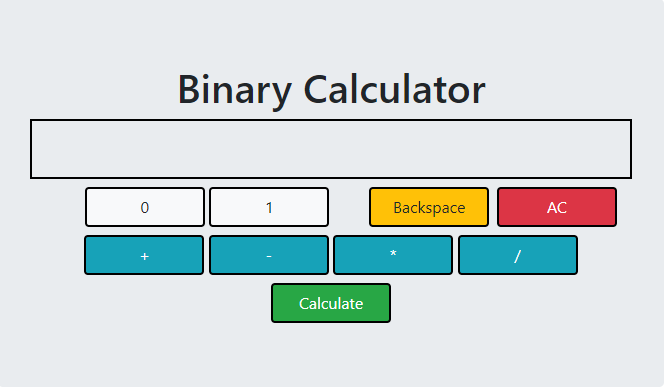Open in App
Not now

# How to Create a Binary Calculator using HTML, CSS and JavaScript ?

• Difficulty Level : Hard
• Last Updated : 16 Jan, 2023

HTML or HyperText Markup Language along with CSS (Cascading Stylesheet) and JavaScript can be used to develop interactive user applications that can perform certain functionalities. Similarly, a binary calculator can be developed using HTML, CSS, and JS altogether.

Binary Calculator performs arithmetic operations on binary numbers. Binary Calculator has a buffer limit of 8 bits. If the result of the arithmetic operation exceeds 8 bits then the extraneous bits are truncated. The arithmetic operations are accomplished using JavaScript functions.

The application consists of a display screen where the user input as well as the result of the arithmetic operation is displayed. Two buttons 0 and 1 are used to take input. The + , – , * , / and Calculate buttons are used to perform an arithmetic operation on the input.

The Calculate button is binded with a JavaScript function calculate(). When the Calculate button is clicked the calculate() function is triggered and the HTML within the ‘output’ division is parsed. The first number and second number are obtained by splitting the string and finally, they are converted into integers using parseInt(). The method parseInt() takes two arguments of which the first is the string to be converted to an integer and the second is the base value which is 2 or binary in this case.

Arithmetic operations are performed depending on the addition, subtraction, multiplication, or division operator chosen by the user. The input() function receives the input from the user and displays it on the screen. The backspace() function deletes the last character of the string displayed. The cls() function clears the display screen. The following code snippets implement a binary calculator.

Example: When an input is given by the user, the input remains within the ‘output’ division in the form of HTML. A global variable scr is declared which can be accessed by all the JavaScript functions. When any input is given it is stored in the scr variable. When the Calculate button is clicked the string stored in the scr variable is searched for the presence of an operator using indexOf() method which returns the index of the operator if found else returns -1. If the operator is present the string stored in scr variable is split at the operator symbol (+,-,*,/), and the strings are stored in the num array. Since the input is in String format it must be converted to binary integer format to perform the calculations. The strings are parsed using parseint(str,base) method where str is the string to be converted and the base is the base of the number (here binary base = 2). After the binary conversion, the specified arithmetic operation is performed and the result is again stored in scr variable and displayed in the ‘output’ division.

## html

 ```<``html``>``    ``<``head``>``        ``<``meta` `charset``=``"utf-8"` `/>``        ``<``title``>Binary Calculator``       ` `        ````        ``<``link` `rel``=``"stylesheet"` `              ``href``=``"https://cdn.jsdelivr.net/npm/bootstrap@4.5.3/dist/css/bootstrap.min.css"` `              ``integrity``=``"sha384-TX8t27EcRE3e/ihU7zmQxVncDAy5uIKz4rEkgIXeMed4M0jlfIDPvg6uqKI2xXr2"` `              ``crossorigin``=``"anonymous"` `/>``    ```` ` `    ``<``body``>``        ``<``div` `class``=``"container"``>``            ``<``div` `class``=``"jumbotron"``>``                ``<``h1``>Binary Calculator``                ``<``div` `id``=``"output"``>``                ``<``div` `class``=``"container mt-2"``>``                    ``<``div` `class``=``"row"``>``                        ``<``div` `class``=``"col-12"``>``                            ``<``button` `type``=``"button"` `                                    ``class``=``"btn btn-light"` `                                    ``onclick``=``"input('0')"``>``                                      ``0``                            ``<``button` `type``=``"button"` `                                    ``class``=``"btn btn-light"` `                                    ``onclick``=``"input('1')"``>``                                      ``1``                            ``<``button` `type``=``"button"` `                                    ``class``=``"btn btn-danger float-right ml-2"` `                                    ``onclick``=``"cls()"``>``                                      ``AC``                            ``<``button` `type``=``"button"` `                                    ``class``=``"btn btn-warning float-right"` `                                    ``onclick``=``"backspace()"``>``                                      ``Backspace``                        ````                    ````                    ``<``div` `class``=``"row mt-2"``>``                        ``<``div` `class``=``"col-12"``>``                            ``<``button` `type``=``"button"` `                                    ``class``=``"btn btn-info"` `                                    ``onclick``=``"input('+')"``>+``                            ``<``button` `type``=``"button"``                                    ``class``=``"btn btn-info"` `                                    ``onclick``=``"input('-')"``>-``                            ``<``button` `type``=``"button"` `                                    ``class``=``"btn btn-info"` `                                    ``onclick``=``"input('*')"``>*``                            ``<``button` `type``=``"button"` `                                    ``class``=``"btn btn-info"` `                                    ``onclick``=``"input('/')"``>/``                        ````                    ````                    ``<``div` `class``=``"row mt-2"``>``                        ``<``div` `class``=``"col-12"``>``                            ``<``button` `type``=``"button"` `                                    ``class``=``"btn btn-success"` `                                    ``onclick``=``"calculate()"``>Calculate``                        ````                    ````                ````            ````        ````        ````        ``<``script` `src``=``"https://code.jquery.com/jquery-3.5.1.slim.min.js"` `                ``integrity``=``"sha384-DfXdz2htPH0lsSSs5nCTpuj/zy4C+OGpamoFVy38MVBnE+IbbVYUew+OrCXaRkfj"` `                ``crossorigin``=``"anonymous"``>``        ``<``script` `src``=``"https://cdn.jsdelivr.net/npm/bootstrap@4.5.3/dist/js/bootstrap.bundle.min.js"` `                ``integrity``=``"sha384-ho+j7jyWK8fNQe+A12Hb8AhRq26LrZ/JpcUGGOn+Y7RsweNrtN/tE3MoK7ZeZDyx"` `                ``crossorigin``=``"anonymous"``>``    `````

## CSS

 `.jumbotron {``    ``width``: ``60%``;``    ``margin``: ``auto``;``    ``text-align``: ``center``;``}`` ` `#output {``    ``border``: ``2px` `solid` `black``;``    ``min-height``: ``60px``;``    ``text-align``: ``right``;``    ``font-weight``: ``bold``;``    ``font-size``: ``20px``;``}`` ` `.btn {``    ``min-width``: ``120px``;``    ``border``: ``2px` `solid` `black``;``}`

## Javascript

 `var` `scr = ``""``; ``//declared as global v``function` `calculate() {``    ``if` `(scr.indexOf(``"+"``) != -1) {``        ``// If + is present in the string``        ``// String obtained from scr is split``        ``var` `num = scr.split(``"+"``);`` ` `        ``// The splitted string stores in num array``        ``var` `x = parseInt(num, 2);`` ` `        ``// The num and num are the two binary ``        ``// numbers resp``        ``var` `y = parseInt(num, 2);``        ``var` `sum = x + y;``        ``var` `ans = sum.toString(2);``    ``} ``else` `if` `(scr.indexOf(``"-"``) != -1) {`` ` `        ``// If - is present in the string``        ``var` `num = scr.split(``"-"``);``        ``var` `x = parseInt(num, 2);``        ``var` `y = parseInt(num, 2);``        ``var` `sub = x - y;``        ``var` `ans = sub.toString(2);``    ``} ``else` `if` `(scr.indexOf(``"*"``) != -1) {`` ` `        ``// If * is present in the string``        ``var` `num = scr.split(``"*"``);``        ``var` `x = parseInt(num, 2);``        ``var` `y = parseInt(num, 2);``        ``var` `mul = x * y;``        ``var` `ans = mul.toString(2);``    ``} ``else` `if` `(scr.indexOf(``"/"``) != -1) {`` ` `        ``// If / is present in the string``        ``var` `num = scr.split(``"/"``);``        ``var` `x = parseInt(num, 2);``        ``var` `y = parseInt(num, 2);``        ``var` `div = x / y;``        ``var` `ans = div.toString(2);``    ``}``    ``scr = ans;``    ``document.getElementById(``"output"``).innerHTML = scr;`` ` `    ``function` `input(ch) {``        ``scr += ch;``        ``document.getElementById(``"output"``).innerHTML = scr;`` ` `        ``function` `backspace() {``            ``var` `b = document.getElementById(``"output"``).innerHTML;``            ``scr = b.substring(0, b.length - 1);``            ``document.getElementById(``"output"``).innerHTML = scr;`` ` `            ``function` `cls() {``                ``scr = ``""``;``                ``document.getElementById(``"output"``).innerHTML = scr;``            ``}`My Personal Notes arrow_drop_up